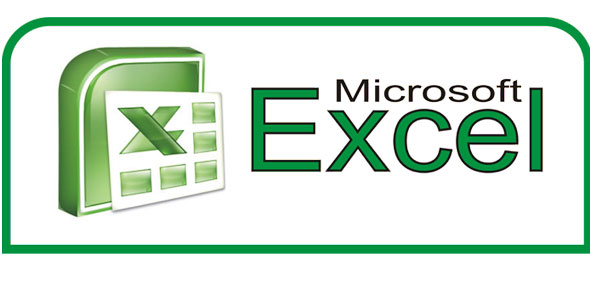# Excel MS

15 Questions | Total Attempts: 40SettingsThis quiz covers beginning terms and knowledge of Excel as well as basic chart information.

• 1.
Which of the following definitions go with Block of Cells?
• A.

The cell with a black line around it showing where data will be added to the spreadsheet

• B.

A group of cells that are adjacent to each other and active

• C.

A group of cells adjacent to each other

• D.

A group of cells collected using the Ctrl key

• 2.
Which of the folloiwing is a cell refernce?
• A.

C15

• B.

5C

• C.

CC

• D.

65,481

• 3.
A label is the same as a value.
• A.

True

• B.

False

• 4.
If you have a value in cell K6, what do you have in the cell?
• A.

Letters

• B.

Words

• C.

Sentences

• D.

Numbers

• 5.
A cell reference is the number of a row then the letter of the column.
• A.

True

• B.

False

• 6.
The file created in Excel is known as a workbook.
• A.

True

• B.

False

• 7.
A file in Excel is made up of 3 ______________ .
• A.

Rows

• B.

Columns

• C.

Worksheets

• D.

Workbooks

• 8.
In Microsoft Excel 2010, Where can you find the open, save, and print commands?
• A.

Home Ribbon

• B.

File Tab

• C.

Workbook Layout Button

• D.

Page Layout Button

• 9.
What do we call the boxes on a spreadsheet?
• A.

Formula bars

• B.

Grids

• C.

Home Boxes

• D.

Cells

• 10.
A _______ is a line of boxes going from left to right.
• A.

Row

• B.

Column

• C.

Formula String

• D.

• 11.
Which of the following formulas could you use to add several cells together?
• A.

=A1*B1*C1

• B.

A1+B1+C1=D1

• C.

=A1+B1+C1

• D.

A1*B1*C1

• 12.
Which of the following formulas could you use to add a range of cells together?
• A.

SUM()

• B.

=(SUM)

• C.

=+SUM()

• D.

=SUM()

• 13.
In excel, a sheet has _________________ rows.
• 14.
In Excel, a sheet has _________________ columns.
• 15.
The Excel program is a part of the ______________ __________________ suite of software.
• A.

Word Perfect

• B.

Microsoft Office

• C.

Microsoft Word

• D.

Microsoft Access

Related Topics